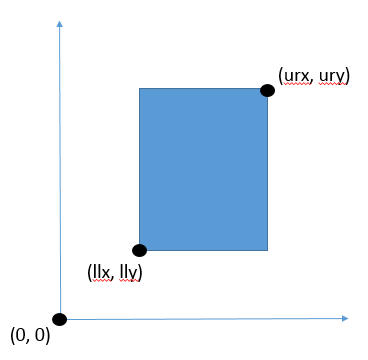iText pdf library

# How should I interpret the coordinates of a rectangle in PDF?

I would like to know more about the coordinates of a Rectangle, in particular:

• lower left X
• lower left Y
• upper right X
• upper right Y

Every time, I get confused about how to make dimensions based on these coordinates to draw rectangle. If possible, can I get a graphical representation briefly about these coordinates positions?

Posted on StackOverflow on Jun 10, 2015 by Nazeerbasha

Before someone can explain what the lower-left X, lower-left Y, upper-right X and upper-right Y of a rectangle are about, you need to know about the coordinate system as explained in the answer to the question "Where is the origin (x,y) of a PDF page?"

The answer to that question contains all the information you need, except for the graphical representation you are asking for. This is a simple representation of the coordinate system:Coordinate system in PDF

The origin of the coordinate system is `(0, 0)`. Positive X values are to the right of the origin, positive Y values are above the origin.

I have drawn a `Rectangle` and indicates where you can find the lower-left corner (with coordinate `(llx, lly)`) and the upper-right corner (with coordinate `(urx, ury)`).

The sides of the rectangle are always in parallel with the X and the Y axis, hence you only need two coordinates to define the rectangle.

Important:

All of the above assumes that you didn't introduce any coordinate transformations, e.g. using the `concatCTM()` or `transform()` method. These methods allow you to change the coordinate system, for instance change the angle between the x and y axis from 90 degrees (the default) to another angle. You can also scale an axis to get a different aspect ratio.

Click this link if you want to see how to answer this question in iText 7.

Contact

## Still have questions?

We're happy to answer your questions. Reach out to us and we'll get back to you shortly.